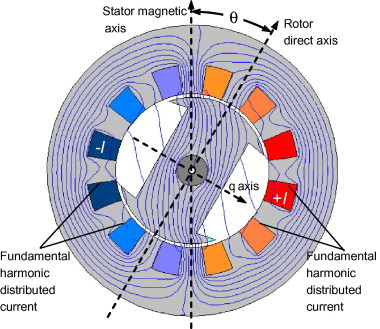## Rotating Magnetic Field Assignment Help

Assignment Help: >> Induction Motors - Rotating Magnetic Field

Rotating Magnetic Field:

We have discussed the general construction of poly phase induction motors. We now will discuss the concept of rotating magnetic field in poly phase induction machines.

Three identical and individual windings, displaced on the stator by 120o and carrying current also displaced 120o in phase or time are needed to establish a constant rotating magnetic field. This magnetic field rotates at synchronous speed (Ns = 120 f/P).

Figure illustrates the stator of a three-phase induction machine. For ease, we will assume the winding concentrated in six (6) slots.Figure: A Generalised Configuration of a Two Pole Three-phase Stator Winding

These concentrated full pitch coils represents the distributed windings generating sinusoidal mmf waves. R, Y and B represent the begin end and R′, Y′ B′ represent the finish end of three phases R, Y and B respectively. We know that the windings of the specific phases are displaced by 120 electrical degrees in the space around the air gap therefore the mmfs of these windings shall also be displaced by 120o in space.

FR, FY and FB represent the mmfs of the three phases, while currents in them are at their positive peak values. Each of the phases current is alternating sinusoid ally with time. As the currents alternate, the mmfs of the three phases shall vary in magnitude and change direction so as to follow modify in current.